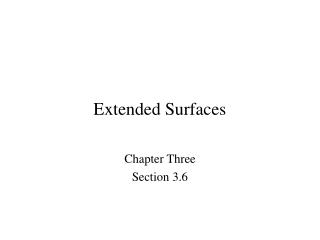Download PresentationExtended Surfaces

Loading in 2 Seconds...

# Extended Surfaces - PowerPoint PPT Presentation

Extended Surfaces. Chapter Three Section 3.6. Nature and Rationale. Nature and Rationale of Extended Surfaces. An extended surface (also know as a combined conduction-convection system or a fin ) is a solid within which heat transfer by conduction is assumed to beI am the owner, or an agent authorized to act on behalf of the owner, of the copyrighted work described.
Download Presentation## Extended Surfaces

An Image/Link below is provided (as is) to download presentation

Download Policy: Content on the Website is provided to you AS IS for your information and personal use and may not be sold / licensed / shared on other websites without getting consent from its author.While downloading, if for some reason you are not able to download a presentation, the publisher may have deleted the file from their server.

- - - - - - - - - - - - - - - - - - - - - - - - - - E N D - - - - - - - - - - - - - - - - - - - - - - - - - -
Presentation Transcript
1. Extended Surfaces Chapter Three Section 3.6

2. Nature and Rationale Nature and Rationale of Extended Surfaces • An extended surface (also know as a combinedconduction-convection system • or a fin) is a solid within which heat transfer by conduction is assumed to be • one dimensional, while heat is also transferred by convection (and/or • radiation) from the surface in a direction transverse to that of conduction. • Why is heat transfer by conduction in the x-direction not, in fact, one- dimensional? • If heat is transferred from the surface to the fluid byconvection, what • surface condition is dictated by the conservation of energy requirement?

3. How does vary with x ? They are particularly beneficial when is small, as for a gas and natural convection. Nature and Rationale (Cont.) • What is the actual functional dependence of the temperature distribution in • the solid? • If the temperature distribution is assumed to be one-dimensional, that is, • T=T(x) , how should the value of T be interpreted for any x location? • When may the assumption of one-dimensional conduction be viewed as an excellent approximation? The thin-fin approximation. • Extended surfaces may exist in many situations but are commonly used as • fins to enhanceheat transfer by increasing the surfacearea available for • convection (and/or radiation). • Some typical fin configurations: Straight fins of (a) uniform and (b) non-uniform cross sections; (c) annular fin, and (d) pin fin of non-uniform cross section.

4. Assuming one-dimensional, steady-state conduction in an extended surface • surface of constantconductivity and uniform cross-sectionalarea , • with negligiblegeneration and radiation , the fin equation • is of the form: (3.62) or, with and the reduced temperature , (3.64) The Fin Equation Fin Equation How is the fin equation derived?

5. Fin Equation • Solutions (Table 3.4): Base (x = 0) condition Tip ( x = L) conditions • Fin Heat Rate:

6. (3.86) Expressions for are provided in Table 3.5 for common geometries. Fin Performance Parameters (3.85) (3.92) Performance Parameters • Fin Efficiency: How is the efficiency affected by the thermal conductivity of the fin? Consider a triangular fin: • Fin Effectiveness: • Fin Resistance:

7. (3.99) Number of fins Area of exposed base (prime surface) (3.101) (3.102) (3.103) Fin Arrays Arrays • Representative arrays of • (a) rectangular and • (b) annular fins. • Total surface area: • Total heat rate: • Overall surface efficiency and resistance:

8. (3.105a) (3.105b) (3.104) Arrays (Cont.) • Equivalent Thermal Circuit : • Effect of Surface Contact Resistance:

9. Problem: Turbine Blade Cooling Problem 3.116: Assessment of cooling scheme for gas turbine blade. Determination of whether blade temperatures are less than the maximum allowable value (1050 °C) for prescribed operating conditions and evaluation of blade cooling rate. Schematic: Assumptions: (1) One-dimensional, steady-state conduction in blade, (2) Constant k, (3) Adiabatic blade tip, (4) Negligible radiation. Analysis: Conditions in the blade are determined by Case B of Table 3.4. (a) With the maximum temperature existing at x=L, Eq. 3.75 yields

10. Problem: Turbine Blade Cooling (cont.) From Table B.1, . Hence, and, subject to the assumption of an adiabatic tip, the operating conditions are acceptable. Eq. 3.76 and Table B.1 yield Hence, Comments: Radiation losses from the blade surface contribute to reducing the blade temperatures, but what is the effect of assuming an adiabatic tip condition? Calculate the tip temperature allowing for convection from the gas.

11. Problem: Chip Heat Sink Problem 3.132: Determination of maximum allowable power for a 20mm x 20mm electronic chip whose temperature is not to exceed when the chip is attached to an air-cooled heat sink with N=11 fins of prescribed dimensions. Schematic: Assumptions: (1) Steady-state, (2) One-dimensional heat transfer, (3) Isothermal chip, (4) Negligible heat transfer from top surface of chip, (5) Negligible temperature rise for air flow, (6) Uniform convection coefficient associated with air flow through channels and over outer surface of heat sink, (7) Negligible radiation.

12. Problem: Chip Heat Sink (cont.) Analysis: (a) From the thermal circuit, From Eqs. (3.103), (3.102), and (3.99)

13. Problem: Chip Heat Sink (cont.) Comments: The heat sink significantly increases the allowable heat dissipation. If it were not used and heat was simply transferred by convection from the surface of the chip with from Part (a) would be replaced by